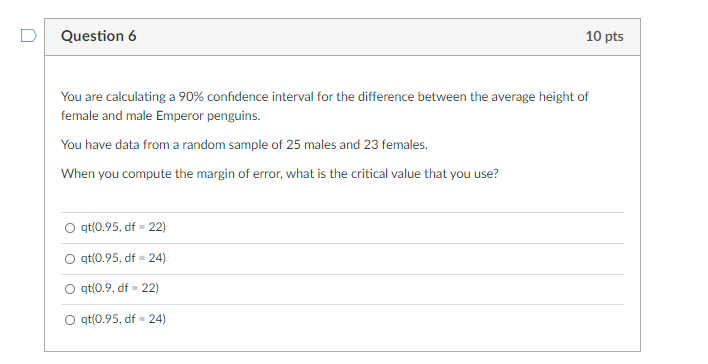# Question Question 6 10 pts You are calculating a 90% confidence interval for the difference between the average height of female and male Emperor penguins. You have data from a random sample of 25 males and 23 females. When you compute the margin of error, what is the critical value that you use? qt(0.95, df -22) O qt(0.95, df -24) O qt(0.9. df - 22) O qt(0.95, df - 24)X1J2CW The Asker · Probability and StatisticsTranscribed Image Text: Question 6 10 pts You are calculating a 90% confidence interval for the difference between the average height of female and male Emperor penguins. You have data from a random sample of 25 males and 23 females. When you compute the margin of error, what is the critical value that you use? qt(0.95, df -22) O qt(0.95, df -24) O qt(0.9. df - 22) O qt(0.95, df - 24)
More
Transcribed Image Text: Question 6 10 pts You are calculating a 90% confidence interval for the difference between the average height of female and male Emperor penguins. You have data from a random sample of 25 males and 23 females. When you compute the margin of error, what is the critical value that you use? qt(0.95, df -22) O qt(0.95, df -24) O qt(0.9. df - 22) O qt(0.95, df - 24)